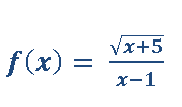Ch.1: Pre-Calc (Part 1)WorksheetSee all chapters
 Ch.1: Pre-Calc (Part 1) 3hrs & 29mins 0% complete Worksheet Ch.2: Pre-Calc (Part 2) 2hrs & 53mins 0% complete Worksheet Ch.3: Pre-Calc (Part 3) 1hr & 40mins 0% complete Worksheet Ch.4: Limits (Part 1) 2hrs & 25mins 0% complete Worksheet Ch.5: Limits (Part 2) 1hr & 53mins 0% complete Worksheet Ch.6: Derivatives (Part 1) 3hrs & 13mins 0% complete Worksheet Ch.7: Derivatives (Part 2) 2hrs & 26mins 0% complete Worksheet Ch.8: Applications of Derivatives (Part 1) 2hrs & 51mins 0% complete Worksheet Ch.9: Applications of Derivatives (Part 2) 2hrs & 1min 0% complete Worksheet Ch.10: Applications of Derivatives (Part 3) 3hrs 0% complete Worksheet Ch.11: Integrals 3hrs & 8mins 0% complete Worksheet

# Computing Domain

See all sections
Sections
Representation of a Function
Word Problems as Functions
Types of Functions
Asymptotes
Rational Functions
Computing Domain
Domain & Range

Concept #1: Intro

Concept #2

Practice: Find the domain for each of the following function,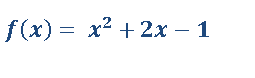Practice: Find the domain for each of the following function,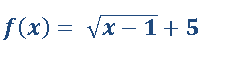Practice: Find the domain for each of the following function,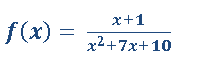Practice: Find the domain for each of the following function,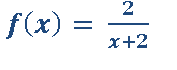Practice: Find the domain for each of the following function,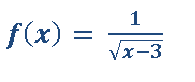Practice: Find the domain for each of the following function,